# 机器学习基础篇：支持向量机（SVM）理论与实践

• 数据点属于该类或
• 数据点不属于该类或

## 复杂的 SVM（非线性可分）

SVM对线性可分数据进行分类有比较好的表现。线性可分数据是任何可以绘制在图形中并且可以使用直线进行分类的数据。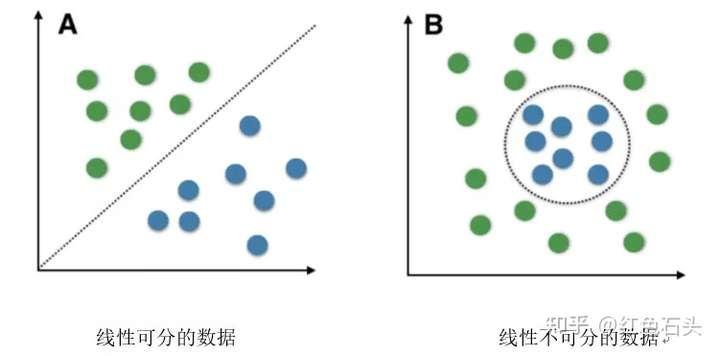Radial BasisFunction Kernel (RBF)：变换后的特征空间中两点之间的相似度是向量与原始输入空间之间距离的指数衰减函数，如下所示。 RBF 是 SVM 中使用的默认内核。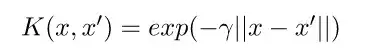1、在有些数据集上表现的比较常好；

2、具有通用性：可以指定不同的内核函数，或者也可以为特定数据类型定义自定义内核；

3、同样适用于高维数据和低维数据。

1、效率（运行时间和内存使用）随着训练集大小的增加而降低；

2、需要标准化输入数据和参数调整，自适应能力弱；

3、没用提供概率估计方法；

4、对做出的预测比较难解释。

## 用python语言实现SVM

# importing scikit learn with make_blobs
from sklearn.datasets.samples_generator import make_blobs

# creating datasets X contarining n_samples
# Y containing two classes
X,Y = make_blobs(n_samples=500, centers=2,
random_state=0, cluster_std=0.40)
import matplotlib.pyplot as plt
# plotting scatters
plt.scatter(X[:,0], X[:, 1], c=Y, s=50, cmap='spring');
plt.show()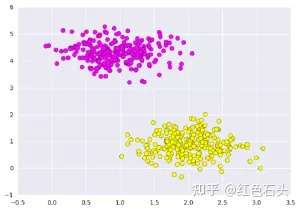SVM不仅是在此处在两个类之间画一条线，而且还要考虑某个给定宽度的线周围的区域。 下面是它的外观示例：

# creating line space between -1 to 3.5
xfit = np.linspace(-1, 3.5)

# plotting scatter
plt.scatter(X[:, 0], X[:, 1], c=Y, s=50, cmap='spring')

# plot a line between the different sets of data
for m, b, d in [(1, 0.65, 0.33), (0.5, 1.6, 0.55), (-0.2, 2.9, 0.2)]:
yfit = m * xfit + b
plt.plot(xfit, yfit, '-k')
plt.fill_between(xfit, yfit - d, yfit + d, edgecolor='none',
color='#AAAAAA', alpha=0.4)

plt.xlim(-1, 3.5);
plt.show()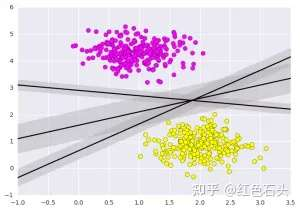# importing required libraries
import numpy as np
import pandas as pd
import matplotlib.pyplot as plt

# reading csv file and extracting class column to y.
a = np.array(x)
y  = a[:,30] # classes having 0 and 1

# extracting two features
x = np.column_stack((x.malignant,x.benign))

# 569 samples and 2 features
x.shape

print (x),(y)


# import support vector classifier
# "Support Vector Classifier"
from sklearn.svm import SVC
clf = SVC(kernel='linear')

# fitting x samples and y classes
clf.fit(x, y)


clf.predict([[120, 990]])

clf.predict([[85, 550]])


array([ 0.])
array([ 1.])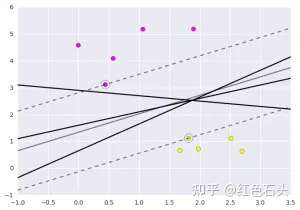https://www.geeksforgeeks.org/introduction-to-support-vector-machines-svm/### 觉得文章有用就打赏一下文章作者

#### 支付宝扫一扫打赏#### 微信扫一扫打赏